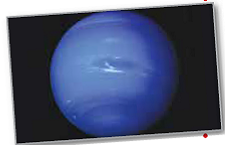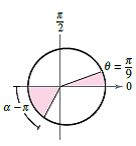Chapter 10.6, Problem 67E

Chapter
Section
Textbook Problem

# Planetary Motion In Exercise 63, the polar equation for the elliptical orbit of Neptune was found. Use the equation and a computer algebra system to perform each of the following.(a) Approximate the area swept out by a ray from then sun to the planet as θ increases from 0 to π / 9 . Use this result to determine the number of years required for the planet to move through this arc when the period of one revolution around the sun is 165 years.(b) By trial and error, approximate the angle α such that the area swept out by a ray from the sun to the planet as θ increases from π to α equals the area found in part (a) (see figure). Does the ray sweep through a larger or smaller angle than in part (a) to generate the same area? Why is this the case?(c) Approximate the distances the planet traveled in parts (a) and (b). Use these distances to approximate the average number of kilometers per year the planet traveled in the two cases.

(a)

To determine

To calculate: The area (approx..) which is swept out by a ray from the sun to the planet as the radians θ increases from 0π9 and use the polar equation of ellipse used in exercise 63 of the chapter and get the result that must be used in order to calculate the number of years required so that the planet to move through this arc when the time period t=165yrs of one revolution around the sun.

Explanation

Given:

Consider the Polar equation of ellipse as follows:

r=4,494,426,03310.0113cosθ

When the time period t=165yrs of one revolution around the sun.

Formula Used:

The Area A of elliptical segment as follows:

A=12αβr2.dθ.

Calculation:

Consider that:

A=0π9r2.dθA=0π9(4,494,426,03310.0113cosθ)2dθ

Use the maple software to evaluate the integral

A=3.591×1018

Let the time period in which the planet will require to move through this arc when period of one revolution is around the sun is 165 years.

Use the Kepler ‘law as follows:

t165=Area of elliptical segmentArea of ellipse.

t165=120π9r2

(b)

To determine

To calculate: The approximate angle α which is swept out by a ray from the sun to the planet as the radians θ increases from πα equals the area found in part (a). Also find if the ray sweep through a larger or smaller angle than in part (a) to calculate the same area.

(c)

To determine

To calculate: The distance the planet travelled in part (a) and part. Use the values of distances to approximate the average number of kilometres in one year that the planet travelled in two cases.

### Still sussing out bartleby?

Check out a sample textbook solution.

See a sample solution

#### The Solution to Your Study Problems

Bartleby provides explanations to thousands of textbook problems written by our experts, many with advanced degrees!

Get Started